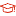UM  > Faculty of Science and Technology
 Affiliated with RC false Status 已發表Published A multigrid method for linear systems arising from time-dependent two-dimensional space-fractional diffusion equations Lin,Xue lei1; Ng,Michael K.2; Sun,Hai Wei12017-02-17 Source Publication Journal of Computational PhysicsISSN 10902716 00219991 Volume 336Pages:69-86 Abstract In this paper, we study a V-cycle multigrid method for linear systems arising from time-dependent two-dimensional space-fractional diffusion equations. The coefficient matrices of the linear systems are structured such that their matrix-vector multiplications can be computed efficiently. The main advantage using the multigrid method is to handle the space-fractional diffusion equations on non-rectangular domains, and to solve the linear systems with non-constant coefficients more effectively. The main idea of the proposed multigrid method is to employ two banded splitting iteration schemes as pre-smoother and post-smoother. The pre-smoother and the post-smoother take banded splitting of the coefficient matrix under the x-dominant ordering and the y-dominant ordering, respectively. We prove the convergence of the proposed two banded splitting iteration schemes in the sense of infinity norm. Results of numerical experiments for time-dependent two-dimensional space-fractional diffusion equations on rectangular, L-shape and U-shape domains are reported to demonstrate that both computational time and iteration number required by the proposed method are significantly smaller than those of the other tested methods. Keyword Banded-splitting Smoother Fractional Diffusion Equation Multigrid Method Non-rectangular Domain Subject Area 偏微分方程数值解 ; 数值代数 ; 计算物理学 DOI 10.1016/j.jcp.2017.02.008 URL View the original Indexed By SCIE Language 英語English WOS Research Area Computer Science ; Physics WOS Subject Computer Science, Interdisciplinary Applications ; Physics, Mathematical WOS ID WOS:000397362800004 Fulltext Access Citation statistics Cited Times [WOS]:29   [WOS Record]     [Related Records in WOS] Document Type Journal article Collection Faculty of Science and Technology Corresponding Author Sun,Hai Wei Affiliation 1.Department of MathematicsUniversity of Macau,Macao2.Department of MathematicsHong Kong Baptist University,Hong Kong First Author Affilication University of Macau Corresponding Author Affilication University of Macau Recommended CitationGB/T 7714 Lin,Xue lei,Ng,Michael K.,Sun,Hai Wei. A multigrid method for linear systems arising from time-dependent two-dimensional space-fractional diffusion equations[J]. Journal of Computational Physics,2017,336:69-86. APA Lin,Xue lei,Ng,Michael K.,&Sun,Hai Wei.(2017).A multigrid method for linear systems arising from time-dependent two-dimensional space-fractional diffusion equations.Journal of Computational Physics,336,69-86. MLA Lin,Xue lei,et al."A multigrid method for linear systems arising from time-dependent two-dimensional space-fractional diffusion equations".Journal of Computational Physics 336(2017):69-86.
 Files in This Item: There are no files associated with this item.
 Related Services Recommend this item Bookmark Usage statistics Export to Endnote Google Scholar Similar articles in Google Scholar [Lin,Xue lei]'s Articles [Ng,Michael K.]'s Articles [Sun,Hai Wei]'s Articles Baidu academic Similar articles in Baidu academic [Lin,Xue lei]'s Articles [Ng,Michael K.]'s Articles [Sun,Hai Wei]'s Articles Bing Scholar Similar articles in Bing Scholar [Lin,Xue lei]'s Articles [Ng,Michael K.]'s Articles [Sun,Hai Wei]'s Articles Terms of Use No data! Social Bookmark/Share